Detection of peaks in data¶

Marcos Duarte
Laboratory of Biomechanics and Motor Control (http://demotu.org/)
Federal University of ABC, Brazil

One way to detect peaks (local maxima) or valleys (local minima) in data is to use the property that a peak (or valley) must be greater (or smaller) than its immediate neighbors. The function detect_peaks.py (code at the end of this notebook) detects peaks (or valleys) based on this feature and other characteristics. The function signature is:

ind = detect_peaks(x, mph=None, mpd=1, threshold=0, edge='rising', kpsh=False, valley=False, show=False, ax=None)

The parameters mph, mpd, and threshold follow the convention of the Matlab function findpeaks.m.
Let's see how to use detect_peaks.py; first let's import the necessary Python libraries and configure the environment:

In :
import numpy as np
import matplotlib.pyplot as plt
%matplotlib inline
import sys
sys.path.insert(1, r'./../functions')  # add to pythonpath
from detect_peaks import detect_peaks

Running the function examples:

In :
>>> from detect_peaks import detect_peaks
>>> x = np.random.randn(100)
>>> x[60:81] = np.nan
>>> # detect all peaks and plot data
>>> ind = detect_peaks(x, show=True)
>>> print(ind)

>>> x = np.sin(2*np.pi*5*np.linspace(0, 1, 200)) + np.random.randn(200)/5
>>> # set minimum peak height = 0 and minimum peak distance = 20
>>> detect_peaks(x, mph=0, mpd=20, show=True)

>>> x = [0, 1, 0, 2, 0, 3, 0, 2, 0, 1, 0]
>>> # set minimum peak distance = 2
>>> detect_peaks(x, mpd=2, show=True)

>>> x = np.sin(2*np.pi*5*np.linspace(0, 1, 200)) + np.random.randn(200)/5
>>> # detection of valleys instead of peaks
>>> detect_peaks(x, mph=-1.2, mpd=20, valley=True, show=True)

>>> x = [0, 1, 1, 0, 1, 1, 0]
>>> # detect both edges
>>> detect_peaks(x, edge='both', show=True)

>>> x = [-2, 1, -2, 2, 1, 1, 3, 0]
>>> # set threshold = 2
>>> detect_peaks(x, threshold = 2, show=True)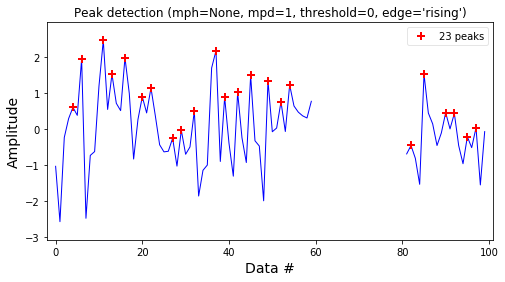[ 4  6 11 13 16 20 22 27 29 32 37 39 42 45 49 52 54 82 85 90 92 95 97]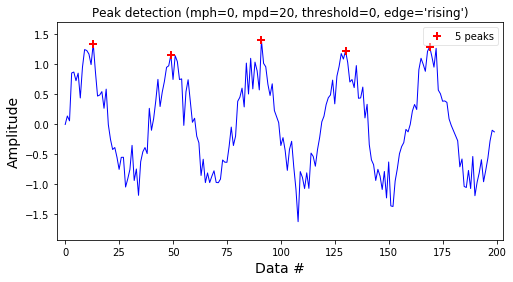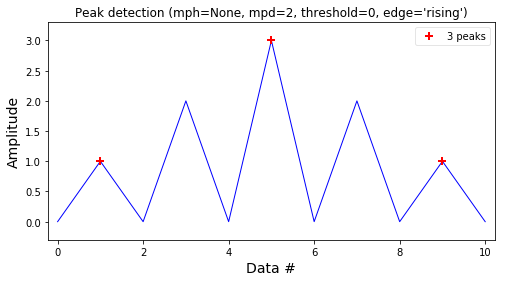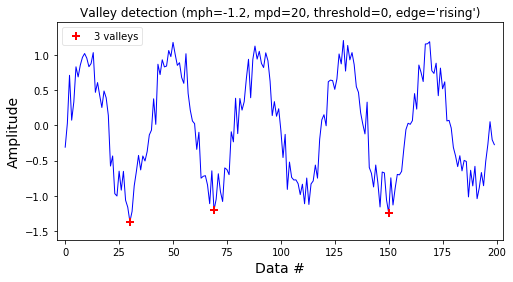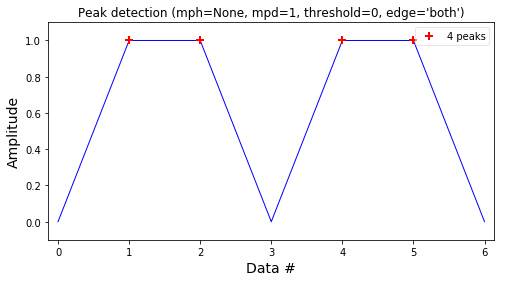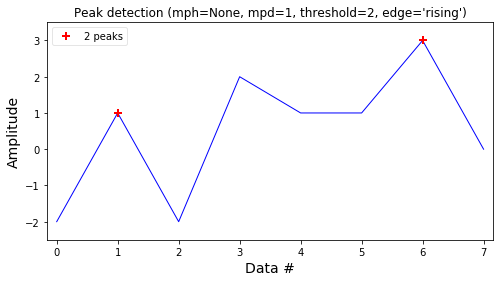Out:
array([1, 6])

Function performance¶

The function detect_peaks.py is relatively fast but the parameter minimum peak distance (mpd) slows down the function if the data has several peaks (>1000). Try to decrease the number of peaks by tuning the other parameters or smooth the data before calling this function with several peaks in the data.
Here is a simple test of its performance:

In :
x = np.random.randn(10000)
ind = detect_peaks(x)
print('Data with %d points and %d peaks\n' %(x.size, ind.size))
print('Performance (without the minimum peak distance parameter):')
print('detect_peaks(x)')
%timeit detect_peaks(x)
print('\nPerformance (using the minimum peak distance parameter):')
print('detect_peaks(x, mpd=10)')
%timeit detect_peaks(x, mpd=10)
Data with 10000 points and 3356 peaks

Performance (without the minimum peak distance parameter):
detect_peaks(x)
91.9 µs ± 1.94 µs per loop (mean ± std. dev. of 7 runs, 10000 loops each)

Performance (using the minimum peak distance parameter):
detect_peaks(x, mpd=10)
7.71 ms ± 30.2 µs per loop (mean ± std. dev. of 7 runs, 100 loops each)

Function detect_peaks.py¶

In [ ]:
"""Detect peaks in data based on their amplitude and other features."""

from __future__ import division, print_function
import numpy as np

__author__ = "Marcos Duarte, https://github.com/demotu/BMC"
__version__ = "1.0.5"

def detect_peaks(x, mph=None, mpd=1, threshold=0, edge='rising',
kpsh=False, valley=False, show=False, ax=None):

"""Detect peaks in data based on their amplitude and other features.

Parameters
----------
x : 1D array_like
data.
mph : {None, number}, optional (default = None)
detect peaks that are greater than minimum peak height (if parameter
valley is False) or peaks that are smaller than maximum peak height
(if parameter valley is True).
mpd : positive integer, optional (default = 1)
detect peaks that are at least separated by minimum peak distance (in
number of data).
threshold : positive number, optional (default = 0)
detect peaks (valleys) that are greater (smaller) than threshold
in relation to their immediate neighbors.
edge : {None, 'rising', 'falling', 'both'}, optional (default = 'rising')
for a flat peak, keep only the rising edge ('rising'), only the
falling edge ('falling'), both edges ('both'), or don't detect a
flat peak (None).
kpsh : bool, optional (default = False)
keep peaks with same height even if they are closer than mpd.
valley : bool, optional (default = False)
if True (1), detect valleys (local minima) instead of peaks.
show : bool, optional (default = False)
if True (1), plot data in matplotlib figure.
ax : a matplotlib.axes.Axes instance, optional (default = None).

Returns
-------
ind : 1D array_like
indeces of the peaks in x.

Notes
-----
The detection of valleys instead of peaks is performed internally by simply
negating the data: ind_valleys = detect_peaks(-x)

The function can handle NaN's

See this IPython Notebook _.

References
----------
..  http://nbviewer.ipython.org/github/demotu/BMC/blob/master/notebooks/DetectPeaks.ipynb

Examples
--------
>>> from detect_peaks import detect_peaks
>>> x = np.random.randn(100)
>>> x[60:81] = np.nan
>>> # detect all peaks and plot data
>>> ind = detect_peaks(x, show=True)
>>> print(ind)

>>> x = np.sin(2*np.pi*5*np.linspace(0, 1, 200)) + np.random.randn(200)/5
>>> # set minimum peak height = 0 and minimum peak distance = 20
>>> detect_peaks(x, mph=0, mpd=20, show=True)

>>> x = [0, 1, 0, 2, 0, 3, 0, 2, 0, 1, 0]
>>> # set minimum peak distance = 2
>>> detect_peaks(x, mpd=2, show=True)

>>> x = np.sin(2*np.pi*5*np.linspace(0, 1, 200)) + np.random.randn(200)/5
>>> # detection of valleys instead of peaks
>>> detect_peaks(x, mph=-1.2, mpd=20, valley=True, show=True)

>>> x = [0, 1, 1, 0, 1, 1, 0]
>>> # detect both edges
>>> detect_peaks(x, edge='both', show=True)

>>> x = [-2, 1, -2, 2, 1, 1, 3, 0]
>>> # set threshold = 2
>>> detect_peaks(x, threshold = 2, show=True)

Version history
---------------
'1.0.5':
The sign of mph is inverted if parameter valley is True

"""

x = np.atleast_1d(x).astype('float64')
if x.size < 3:
return np.array([], dtype=int)
if valley:
x = -x
if mph is not None:
mph = -mph
# find indices of all peaks
dx = x[1:] - x[:-1]
# handle NaN's
indnan = np.where(np.isnan(x))
if indnan.size:
x[indnan] = np.inf
dx[np.where(np.isnan(dx))] = np.inf
ine, ire, ife = np.array([[], [], []], dtype=int)
if not edge:
ine = np.where((np.hstack((dx, 0)) < 0) & (np.hstack((0, dx)) > 0))
else:
if edge.lower() in ['rising', 'both']:
ire = np.where((np.hstack((dx, 0)) <= 0) & (np.hstack((0, dx)) > 0))
if edge.lower() in ['falling', 'both']:
ife = np.where((np.hstack((dx, 0)) < 0) & (np.hstack((0, dx)) >= 0))
ind = np.unique(np.hstack((ine, ire, ife)))
# handle NaN's
if ind.size and indnan.size:
# NaN's and values close to NaN's cannot be peaks
ind = ind[np.in1d(ind, np.unique(np.hstack((indnan, indnan-1, indnan+1))), invert=True)]
# first and last values of x cannot be peaks
if ind.size and ind == 0:
ind = ind[1:]
if ind.size and ind[-1] == x.size-1:
ind = ind[:-1]
# remove peaks < minimum peak height
if ind.size and mph is not None:
ind = ind[x[ind] >= mph]
# remove peaks - neighbors < threshold
if ind.size and threshold > 0:
dx = np.min(np.vstack([x[ind]-x[ind-1], x[ind]-x[ind+1]]), axis=0)
ind = np.delete(ind, np.where(dx < threshold))
# detect small peaks closer than minimum peak distance
if ind.size and mpd > 1:
ind = ind[np.argsort(x[ind])][::-1]  # sort ind by peak height
idel = np.zeros(ind.size, dtype=bool)
for i in range(ind.size):
if not idel[i]:
# keep peaks with the same height if kpsh is True
idel = idel | (ind >= ind[i] - mpd) & (ind <= ind[i] + mpd) \
& (x[ind[i]] > x[ind] if kpsh else True)
idel[i] = 0  # Keep current peak
# remove the small peaks and sort back the indices by their occurrence
ind = np.sort(ind[~idel])

if show:
if indnan.size:
x[indnan] = np.nan
if valley:
x = -x
if mph is not None:
mph = -mph
_plot(x, mph, mpd, threshold, edge, valley, ax, ind)

return ind

def _plot(x, mph, mpd, threshold, edge, valley, ax, ind):
"""Plot results of the detect_peaks function, see its help."""
try:
import matplotlib.pyplot as plt
except ImportError:
print('matplotlib is not available.')
else:
if ax is None:
_, ax = plt.subplots(1, 1, figsize=(8, 4))

ax.plot(x, 'b', lw=1)
if ind.size:
label = 'valley' if valley else 'peak'
label = label + 's' if ind.size > 1 else label
ax.plot(ind, x[ind], '+', mfc=None, mec='r', mew=2, ms=8,
label='%d %s' % (ind.size, label))
ax.legend(loc='best', framealpha=.5, numpoints=1)
ax.set_xlim(-.02*x.size, x.size*1.02-1)
ymin, ymax = x[np.isfinite(x)].min(), x[np.isfinite(x)].max()
yrange = ymax - ymin if ymax > ymin else 1
ax.set_ylim(ymin - 0.1*yrange, ymax + 0.1*yrange)
ax.set_xlabel('Data #', fontsize=14)
ax.set_ylabel('Amplitude', fontsize=14)
mode = 'Valley detection' if valley else 'Peak detection'
ax.set_title("%s (mph=%s, mpd=%d, threshold=%s, edge='%s')"
% (mode, str(mph), mpd, str(threshold), edge))
# plt.grid()
plt.show()